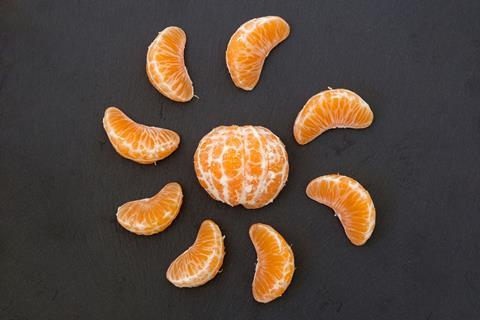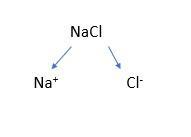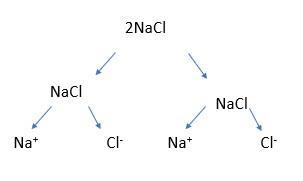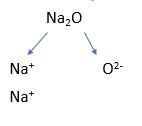Adam Boxer shows us how to sequence and segment the topic of ionic equations effectivelySequencing and segmenting a topic is key to students understanding and remembering information

Sequencing and segmenting is one of the most powerful concepts in a science teacher’s arsenal. In short, it determines the order in which topics are taught, and where you choose to break them up for students to have an opportunity to practise. If your sequencing is off, students won’t understand your explanation. If your segmenting is off, they won’t remember your explanation. Below is an example of how you might tackle this for one of the most challenging topics to teach at 14–16 chemistry: construction of ionic equations.

We could start here with the most common problems students have when constructing these equations and use those to work backwards. If we can isolate each difficulty, then we can teach them explicitly in turn and allow students’ skills to gradually build. In my experience, students have difficulty with:

• Writing an ionic compound as ions.
• Confusing charges with subscript numbers.
• Turning subscript numbers into coefficients.
• Turning coefficients into subscript numbers.
• Appreciation of which substances can form ions and which ones cannot.

To address these, we can sequence and segment as follows:

### 1. Compounds into ions

First, give students some recall questions for the formation of a compound like sodium chloride along the lines of:

• Draw the full atomic structure/electronic configuration of sodium and chlorine.
• Use arrows to show what happens when they bond ionically.
• Draw both ions out.
• Write the formulas for the ions.

This enables you to test prerequisite knowledge, give your students the opportunity for retrieval practice and help your students see the connections between different topics.

Then show students a simple graphical way of writing the compound as ions:Do another example, then give students some examples to do themselves, each with 1:1 ratios like calcium oxide or aluminium nitride (see attached resource for an example sequence).

Do another example, then give students some examples to do themselves, each with 1:1 ratios like calcium oxide or aluminium nitride (see the accompanying resource for an example sequence: rsc.li/3aZ6LeZ).

### 2. Stoichiometry

Next, discuss with students what it would look like with 2NaCl. Draw it out again:And explain that you would write this as 2Na+ + 2Cl-

Again, give students the opportunity to practise this with more 1:1 examples but with a range of stoichiometric numbers.

### 3. Subscript

Now is the time to introduce subscript numbers, perhaps using sodium oxide as an example, which is written as 2Na+ + O2-:Give students some more to practise, then pause and show them an example like 2Na2O to marry this step with the previous step. Give them a lot more practice, mixing examples with different ionic ratios and stoichiometric numbers. You can also add written formulas as well like ‘sodium sulfide’ to help them realise how interconnected the topics are and that you can go from formulas to ions and from ions to formulas.

### 4. Polyatomic ions

You will also want to introduce polyatomic ions like sulfate, hydroxide and carbonate. As above, model a few examples, then give students an opportunity to practise, keeping your first examples varying only slightly, for example: sodium hydroxide, sodium sulfate, sodium carbonate, aluminium hydroxide, aluminium sulfate etc.

### 5. Covalent

You will have taught your students that covalent substances are distinct from ionic ones and therefore do not have ions in them. Use a simple example like chlorine and refer back to your previous explanations showing that chlorine does not split into Cl- and Cl+ ions. Then give students a further practice set similar to the one above but interspersed with covalent examples (don’t forget to vary stoichiometry, ionic ratios and polyatomic ions). As above, you can introduce words like ‘graphite’, ‘silicon dioxide’ and ‘methane’.

### 6. Ionic conditions

Covalent substances are an easier introduction to ‘substances which do not split into ions’ than solid ionic substances. Use them as a springboard for the next step saying something like ‘in truth it isn’t just covalent substances that don’t split into ions, solid ones don’t either …’ going back to your previous lessons about giant ionic lattices and electrostatic interactions. Explicitly model to students that it is only (l) and (aq) ionic substances that split into ions: nothing else does. Now give students a further practice set, including non-1:1 ratios, varied stoichiometry, interspersed covalent examples and state symbols.

### 7. Acids

Before starting ionic equations, most schemes of work will have introduced acids and their various reactions. You can now go back to the three major acids and discuss how they also split into ions, despite them looking at first glance like covalent substances. By this point, your students should not be confused as to why H2SO4 forms two H+ ions.

### 8. Ionic equations

Your students are finally ready to tackle ionic equations. I would normally do a few similar and simple ones (metal + acid), explain the elimination of spectator ions and then give students the opportunity to progress through some varied practice. A practice sequence might look like the following:

1. Metal + acid, given full balanced symbol equation
2. Metal + acid, given full symbol equation
3. Metal + acid, given partial word equation (reactants)
4. Metal + acid, given partial word equation (products)
5. Repeat a–d but for metal hydroxide + acid
6. A couple of questions on metal oxide and metal carbonate + acid
7. Free practice with interspersed examples (including things like halogen displacements)

It may seem like a long way to go about it, but you can almost guarantee that they will be better chemists for it, not only in terms of their ability to construct ionic equations, but also to understand why they are the way they are, where all the rules come from and how chemistry’s interconnectedness is perhaps its most defining – and beautiful – characteristic in school science.